#### Write2

##### Description

Write2[file, val1 = expr1, val2 = expr2, ...] writes the settings val1 = expr1, val2 = expr2 in sequence followed by a newline, to the specified output file. Setting the option FormatType of Write2 to FortranForm results in FORTRAN syntax output.

The continuation character for FORTRAN output can be controlled by changing \$FortranContinuationCharacter.

``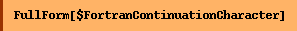``
`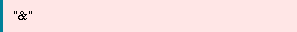`
``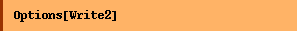``
`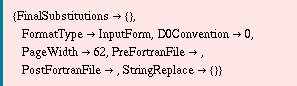`
``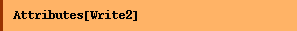``
`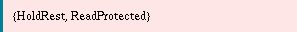`

##### Examples
``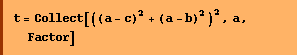``
`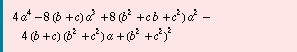`
``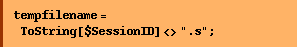``

This writes the assignment r=t to a file.

``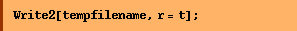``

This shows the contents of the file.

``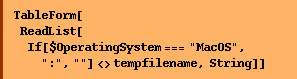``
 r = ( 4*a^4 - 8*a^3*(b + c) - 4*a*(b + c)*(b^2 + c^2) + (b^2 + c^2)^2 + 8*a^2*(b^2 + b*c + c^2) );
``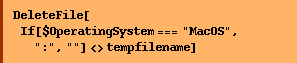``
``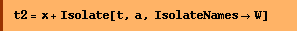``
`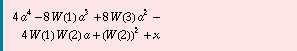`
``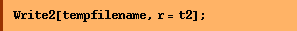``
````
 W = (b + c ); W = (b^2 + c^2 ); W = (b^2 + b*c + c^2 ); r = ( 4*a^4 + x - 8*a^3*HoldForm[W] - 4*a*HoldForm[W]*HoldForm[W] + HoldForm[W]^2 + 8*a^2*HoldForm[W] );
````

This is how to write out the expression t2 in Fortran format.

``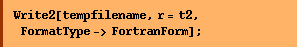``
````
 W(1)= b + c W(2)= b**2 + c**2 W(3)= b**2 + b*c + c**2 r = 4*a**4 + x - 8*a**3*W(1) - 4*a*W(1)*W(2) + W(2)**2 + &  8*a**2*W(3)
``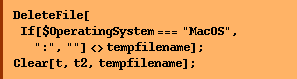``

Converted from the Mathematica notebook Write2.nb